﻿ Available Options | TT-01 Types | Miki Pulley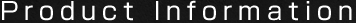# Torque Limiters TT(01) Types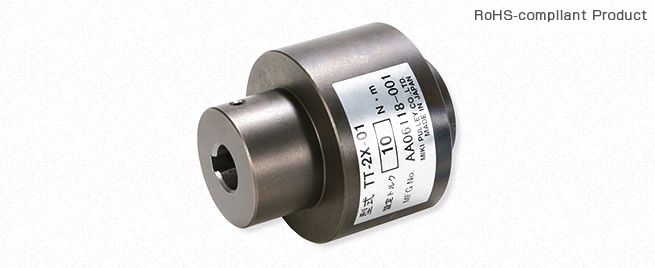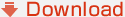## Options of Torque Limiters TT(01) Types

### Optional Signal Pin

Unattended or remotely controlled machines and equipment require equipment that detects an overload and automatically switches off the power or sounds a warning alarm.
An overload can be detected by connecting the signal pin to the torque tender.
When an overload is detected, the input side and the output side are disconnected and the cam mechanism of the torque tender hub pushes the signal pin out in the radial direction. This can be used to switch off the power or sound a warning alarm.
Be sure to use the housing as the input side.
The standard product cannot be modified to connect the signal pin. If you need to
connect the signal pin, add "-P" to the end of the model when you order the product.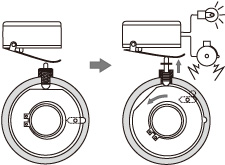Unit (mm)
Size M Y R N
1X 24 1.5 6.5 5.5
2 29 2.5 5 4.5
2X 36 2.5 5 4.5
3 43 2.5 5 4.5
3X 42 2.5 5 4.5
4X 55 2.5 5 2

Standard Hole-Drilling Standards

*Set screws are included with the product.
*For standard dimensions for bore drilling other than those given here, contact Miki Pulley.
(A bore may not be able to be drilled for some hub sizes.)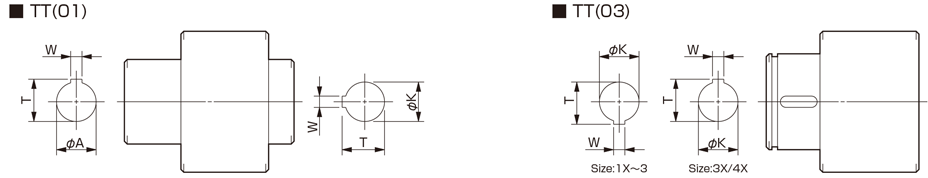Unit [mm]
Models compliant with the old JIS standard (class 2) JIS B 1301 1959 Models compliant with the new JIS standard (H9) JIS B 1301 1996 Models compliant with the motor standard JIS C 4210 2001
Nominal bore
diameter
Bore
diameter
[oA/oK]
Keyway
width
[W]
Keyway
height
[T]
Nominal bore
diameter
Bore
diameter
[oA/oK]
Keyway
width
[W]
Keyway
height
[T]
Nominal bore
diameter
Bore
diameter
[oA/oK]
Keyway
width
[W]
Keyway
height
[T]
Tolerance
H7
Tolerance
E9
- Tolerance
H7
Tolerance
H9
- Tolerance
G7
-
8 8+0.0150 - - - - - - - - - -
10 10+0.0150 4+0.050+0.020 11.5+0.50 10H 10+0.0150 4+0.0300 11.8+0.50 - - - -
11 11+0.0180 4+0.050+0.020 12.5+0.50 11H 11+0.0180 4+0.0300 12.8+0.50 - - - -
12 12+0.0180 4+0.050+0.020 13.5+0.50 12H 12+0.0180 4+0.0300 13.8+0.50 - - - -
14 14+0.0180 5+0.050+0.020 16.0+0.50 14H 14+0.0180 5+0.0300 16.3+0.50 14N 14+0.024+0.006 5+0.0300 16.0+0.50
15 15+0.0180 5+0.050+0.020 17.0+0.50 15H 15+0.0180 5+0.0300 17.3+0.50 - - - -
16 16+0.0180 5+0.050+0.020 18.0+0.50 16H 16+0.0180 5+0.0300 18.3+0.50 - - - -
18 18+0.0180 5+0.050+0.020 20.0+0.50 18H 18+0.0180 6+0.0300 20.8+0.50 - - - -
19 19+0.0210 5+0.050+0.020 21.0+0.50 19H 19+0.0210 6+0.0300 21.8+0.50 19N 19+0.028+0.007 6+0.0300 21.5+0.50
20 20+0.0210 5+0.050+0.020 22.0+0.50 20H 20+0.0210 6+0.0300 22.8+0.50 - - - -
22 22+0.0210 7+0.061+0.025 25.0+0.50 22H 22+0.0210 6+0.0300 24.8+0.50 - - - -
24 24+0.0210 7+0.061+0.025 27.0+0.50 24H 24+0.0210 8+0.0360 27.3+0.50 24N 24+0.028+0.007 8+0.0360 27.0+0.50
25 25+0.0210 7+0.061+0.025 28.0+0.50 25H 25+0.0210 8+0.0360 28.3+0.50 - - - -
28 28+0.0210 7+0.061+0.025 31.0+0.50 28H 28+0.0210 8+0.0360 31.3+0.50 28N 28+0.028+0.007 8+0.0360 31.0+0.50
30 30+0.0210 7+0.061+0.025 33.0+0.50 30H 30+0.0210 8+0.0360 33.3+0.50 - - - -
32 32+0.0250 10+0.061+0.025 35.5+0.50 32H 32+0.0250 10+0.0360 35.3+0.50 - - - -
35 35+0.0250 10+0.061+0.025 38.5+0.50 35H 35+0.0250 10+0.0360 38.3+0.50 - - - -
38 38+0.0250 10+0.061+0.025 41.5+0.50 38H 38+0.0250 10+0.0360 41.3+0.50 38N 38+0.034+0.009 10+0.0360 41.0+0.50
40 40+0.0250 10+0.061+0.025 43.5+0.50 40H 40+0.0250 12+0.0430 43.3+0.50 - - - -
42 42+0.0250 10+0.061+0.025 45.5+0.50 42H 42+0.0250 12+0.0430 45.3+0.50 42N 42+0.034+0.009 12+0.0430 45.0+0.50
45 45+0.0250 12+0.075+0.032 48.5+0.50 45H 45+0.0250 14+0.0430 48.8+0.50 - - - -
48 48+0.0250 12+0.075+0.032 51.5+0.50 48H 48+0.0250 14+0.0430 51.8+0.50 48N 48+0.034+0.009 14+0.0430 51.5+0.50
50 50+0.0250 12+0.075+0.032 53.5+0.50 50H 50+0.0250 14+0.0430 53.8+0.50 - - - -

﻿